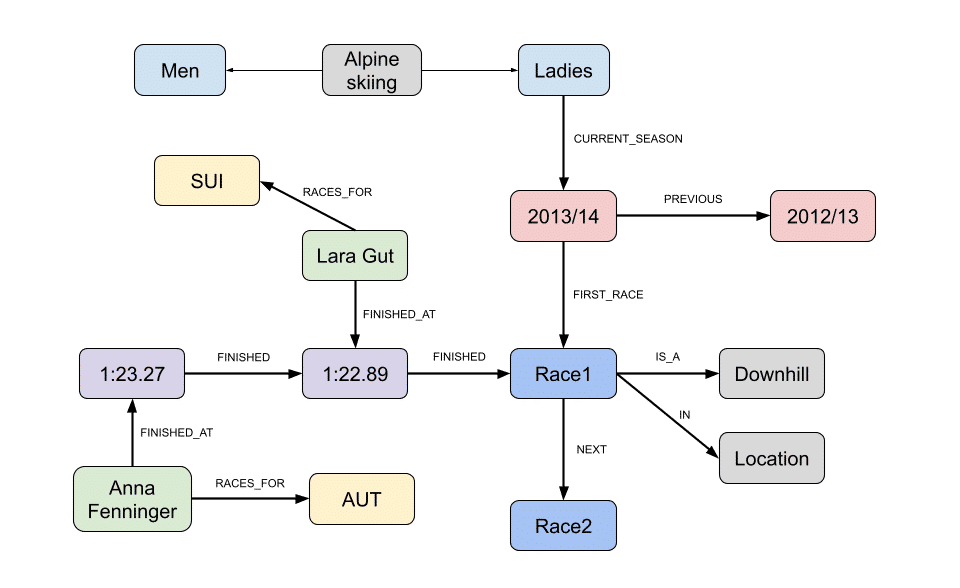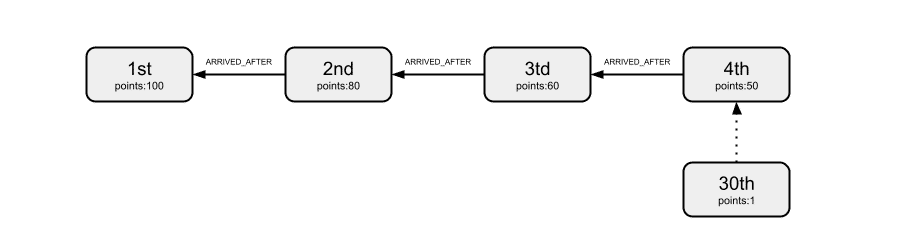# FIS Alpine Skiing Seasons

## Introduction

I was interested on playing with Neo4j with some real data and so I grabbed some from the FIS site and tried to play with it. The outcome is a graph that stores the results of alpine skiing races.

The idea is to have a graph that is easy to navigate: you can start from the Alpine skiing node, then go to Ladies, then to the current season and then to the first race, then to the next race, and so on. Every race has its type (Downhill, Slalom , Giant Slalom , Super G, Super Combined) and location. Athletes are connected to the races through their results. Seasons, races and results are linked lists.

2014 will be the year of the XXII winter olympic games in Sochi. Go Switzerland!

### Graph model## Use cases

``````MATCH (g:Gender)-[:CURRENT_SEASON]->(s:Season)-[*]->(r:Race)
match (r)-[:IS_A]->(t), (r)-[:IN]->(l)
return r.date as date, l.name as location, t.name as type``````

### Ranking by race

``````MATCH (r:Race)
where r.date = '29/11/2013'
match (r)<-[time:FINISHED*]-(f)<-[:FINISHED_AT]-(racer)
with racer, f, time, reduce(totalDiff = 0, n IN time| totalDiff + n.diff) as diff
return size(time) as rank, racer.firstname+" "+racer.lastname as racer, f.time as time, "+"+diff as diff``````

``````MATCH (g:Gender)-[:CURRENT_SEASON]->(s:Season)-[*]->(r:Race)
with r
MATCH (n:Athlete)
MATCH p = allShortestPaths((n)-[:FINISHED_AT|FINISHED*]->(r))
WITH size(relationships(p))-1 as c, p
WITH CASE
WHEN c < 4
THEN 100-((c-1)*20)
WHEN c < 7
THEN 50-((c-4)*5)
WHEN c = 7
THEN 36
WHEN c < 11
THEN 32-((c-8)*3)
WHEN c < 16
THEN 24 -((c-11)*2)
WHEN c < 31
THEN 31-c
ELSE 0  END AS result, p
return distinct(head(nodes(p)).firstname+' '+head(nodes(p)).lastname) as racer , sum(result) as points order by points desc``````

Another way to calculate ranking points

Added a second graph with the ranking points. Every node is a ranking position, with its related points. Doing so, the association between rank and points is no more in the query, but in the database.``````MATCH (g:Gender)-[:CURRENT_SEASON]->(s:Season)-[*]->(r:Race)
with r
MATCH (n:Athlete)
MATCH p = allShortestPaths((n)-[:FINISHED_AT|:FINISHED*]->(r))
WITH length(relationships(p))-1 as c, p
match (rp:Rankpoints)
where rp.label = c+''
return distinct(head(nodes(p)).firstname+' '+head(nodes(p)).lastname) as racer , sum(rp.points) as points  order by points desc``````

``````MATCH (g:Gender)-[:CURRENT_SEASON]->(s:Season)-[*]->(r:Race)
with r
MATCH (n:Athlete), r-[:IS_A]->(t:Type)
Where t.name = 'Downhill'
MATCH p = allShortestPaths((n)-[:FINISHED_AT|:FINISHED*]->(r))
WITH length(relationships(p))-1 as c, p
match (rp:Rankpoints)
where rp.label = c+''
return distinct(head(nodes(p)).firstname+' '+head(nodes(p)).lastname) as racer , sum(rp.points) as points  order by points desc``````

``````MATCH (g:Gender)-[:CURRENT_SEASON]->(s:Season)-[*]->(r:Race)
with r
MATCH (n:Athlete)-[:RACES_FOR]->(country:Country)
MATCH p = allShortestPaths((n)-[:FINISHED_AT|:FINISHED*]->(r))
WITH length(relationships(p))-1 as c, p
match (rp:Rankpoints)
where rp.label = c+''
match e-[:RACES_FOR]->(d)
return distinct(d.name) as country , sum(rp.points) as points  order by points desc``````

### Podiums by athlete ever

Podiums of Lara Gut ever

``````MATCH (n:Athlete),(r:Race)
WHERE n.lastname = 'Gut'
MATCH p = allShortestPaths((n)-[:FINISHED_AT|FINISHED*..4]->(r))
RETURN count(p) as Gut_podiums``````

### Rank by athlete in all races on current season

Rank of Anna Fenninger in all races in current season

``````MATCH (g:Gender)-[:CURRENT_SEASON]->(s:Season)-[*]->(r:Race)
with r
MATCH (n:Athlete)
WHERE n.lastname = 'Fenninger'
MATCH p = allShortestPaths((n)-[:FINISHED_AT|FINISHED*]->(r))
MATCH r-[:IS_A]->(t), r-[:IN]->(c)
return r.date as date, t.name as type, c.name as location, length(relationships(p))-1 as rank``````

### Average ranking by athlete

Average ranking of Anna Fenninger ever

``````MATCH (n:Athlete),(r:Race)
WHERE n.lastname = 'Fenninger'
MATCH p = allShortestPaths((n)-[:FINISHED_AT|FINISHED*]->(r))
MATCH r-[:IS_A]->(t), r-[:IN]->(c)
return avg(length(relationships(p))-1) as avg_rank, "over "+count(p)+" races" as races``````

### Podiums by country ever

Podiums of Austria ever

``````MATCH (n:Country)
WHERE n.name = 'AUT'
MATCH (a:Athlete)-[:RACES_FOR]->(n),(r:Race)
MATCH p = allShortestPaths((a)-[:FINISHED_AT|FINISHED*..4]->(r))
RETURN count(p) as AUT_podiums``````

### How close was a victory

How close to first place was a second place

``````MATCH (n:Athlete),(r:Race)
MATCH p = allShortestPaths((n)-[:FINISHED_AT|FINISHED*..3]->(r))
with filter(x IN relationships(p) WHERE (x.diff < 200 and x.diff <> 0)) as filtered
with filtered as m
match (racer)-[:FINISHED_AT]->()-[m]->()-[:FINISHED]->(r), r-[:IN]->(l), r-[:IS_A]->(t)
return racer.firstname+' '+racer.lastname as racer, r.date as date,l.name as location, t.name as type,"+"+m.diff as diff``````